# High School Physics : Resistivity

## Example Questions

← Previous 1

### Example Question #949 : High School Physics

Ten resistors, each with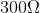resistance, are set up in series. What is their equivalent resistance?

Possible Answers: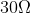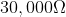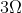Correct answer:Explanation:

For resistors aligned in series, the equivalent resistance is the sum of the individual resistances.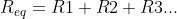Since all the resistors in this problem are equal, we can simplify with multiplication.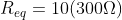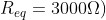### Example Question #950 : High School Physics

What is the total resistance of a parallel circuit with resistors of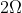,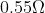, and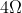?

Possible Answers: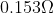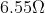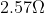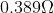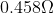Correct answer:Explanation:

The formula for resistance in parallel is: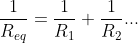We are given the values for each individual resistor, allowing us to solve for the total resistance.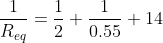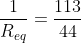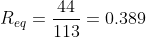### Example Question #91 : Electricity And Magnetism

An electric circuit is set up in series with five resistors. If the resistors remain the same, but the circuit is now set up with the resistors in parallel, how would this affect the total resistance?

Possible Answers:

Resistance would remain unchanged

Resistance would increase substantially

We need to know the numerical values to solve

Resistance would decrease substantially

Correct answer:

Resistance would decrease substantially

Explanation:

Think of resistors as doors, preventing the flow of people (electrons). Imagine the following scenarios: a large group of people are in a room and all try to leave at once. If the five resistors are in series, that's like having all of these people trying to go through all five doors before they can leave. In a circuit, all the electrons in the current mast pass through every resistor.

If the resistors are in parallel, it's like having five separate doors from the room. All of a sudden, the group can leave MUCH faster, encountering less resistance to their flow out of the room. The path of the electrons can split, allowing each particle to pass through only one resistor.

From a formula perspective, the resistors in series are simply summed together to find the equivalent resistance.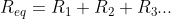In parallel, however, the reciprocals are summed to find the reciprocal equivalent resistance.Adding whole numbers will always give you a much greater result than adding fractions. For the exact same set of resistors, arrangement in series will have a greater total resistance than arrangement in parallel.

### Example Question #92 : Electricity And Magnetism

When current in a circuit crosses a resistor, energy is lost. What form does this lost energy most commonly take?

Possible Answers:

The energy is converted into heat

The energy is converted into light

The energy is not converted; it simply disappears

The energy is converted into sound

The energy is converted into motion

Correct answer:

The energy is converted into heat

Explanation:

In basic resistors, energy lost due to resistance is converted into heat. In some cases, other conversions also take place (such as generation of light in a lightbulb), but heat is still dissipated along with any alternative conversations. Lightbulbs, batteries, and other types of resistors will become hot as current passes through them.

### Example Question #93 : Electricity And Magnetism

You have a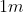long,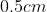diameter copper wire that has an electric current running through it. Which of the following would decrease the wire's overall resistivity?

Possible Answers:

Decreasing the diameter of the wire

None of these

Increasing the length of the wire

Increasing the diameter of the wire

Decreasing the length of the wire

Correct answer:

None of these

Explanation:

The formula for resistance is: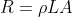Above,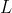is length,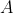is the cross-sectional area of the wire, and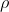is the resistivity of the material, and is a property of that material. The resistivity is constant for a given material, and thus cannot be changed by altering the dimensions of the wire.

### Example Question #94 : Electricity And Magnetism

Two aluminum wires have the same resistance.  If one has twice the length of the other, what is the ratio of the diameter of the longer wire to the diameter of the shorter wire?

Possible Answers: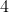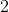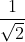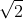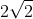Correct answer:Explanation:

Let’s start with the resistivity equation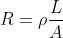The area of a wire is the area of a circle.  So let’s substitute that into our equation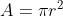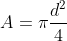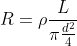This can be simplified to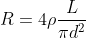Since we know that both resistors have the same resistivity and the same resistance, we can set these equations equal to each other.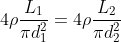Many things fallout which leaves us with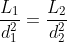We know that the second wire is twice the length as the first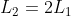So we can substitute this into our equation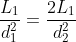The length of the wire drops out of the equation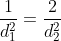Now we can solve for the diameter of the longer wire.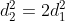Take the square root of both sides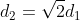Therefore the ratio of the long to the short wire is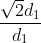Or### Example Question #95 : Electricity And Magnetism

What is the diameter of a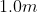length of tungsten wire whose resistance is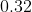ohms?

Possible Answers: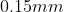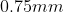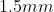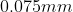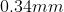Correct answer:Explanation:

We will use the resistivity equation to solve for this.  We know

Length =Resistance =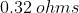ρ of Tungsten =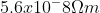The equation for resistivity isWe can rearrange this equation to solve for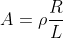In this case the area of the wire is the area of a circle which is equal to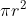We can rearrange this to get the radius by itself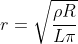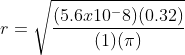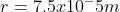To find the diameter we need to multiply this value by 2.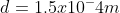### Example Question #21 : Resistors

The resistivity of most common metals __________________ .

Possible Answers:

decreases as the temperature increases

remains constant over wide temperature ranges

varies randomly as the temperature increases

increases as the temperature increases

Correct answer:

increases as the temperature increases

Explanation:

At higher temperature, the atoms are moving more rapidly and are arranged in a less orderly way.  Therefore it is expected that these fast moving atoms are more likely to interfere with the flow of electrons.  If there is more interference in the flow of electrons, then there is a higher resistivity.

### Example Question #22 : Resistors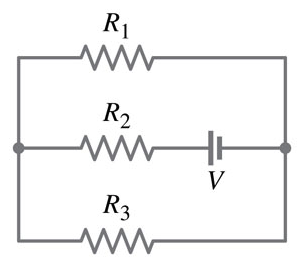Which Resistors are connected in parallel?

Possible Answers: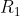and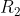All answer choices are correctand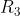None of the answer choices are correctandCorrect answer:andExplanation:

Resistors are in parallel when the electric current passes through two or more branches or connected parts at the same time before it combines again. After the current leaves the battery it travels to. Then the current splits and travels between the other two resistors before the current coming back together and connecting back to the battery.

### Example Question #23 : Resistors

How does adding resistors in parallel affect the overall current of the circuit?

Possible Answers:

The current stays the same

The current increases

The current decreases

Correct answer:

The current increases

Explanation:

Adding resistors in parallel decrease the overall equivalent resistance as they are added using the equation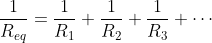Since the overall equivalent resistance is decreased, if the voltage is constant the overall current would increase.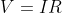Therefore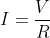This shows the inverse relationship between the two values.

← Previous 1

### All High School Physics Resources